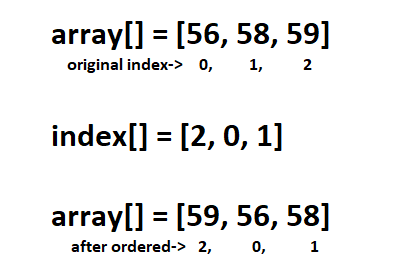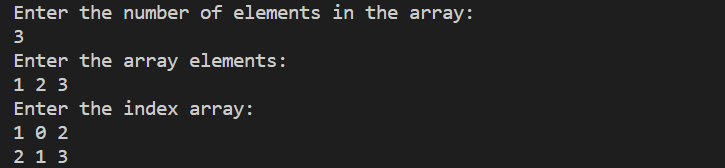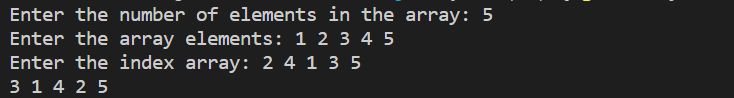New update is available. Click here to update.

# Array Reordering Using Given Indexes

## Introduction

An array data structure, or simply an array, is a data structure in computer science that consists of a collection of items, each of which is identified by at least one array index or key.

In this article, we will learn to reorder an array. To reorder, we will be provided with another array that will contain the value of the indexes. Using these indexes, we have to reorder the original array.

Let’s begin with an introduction to the problem statement.

## Problem Statement

We need to reorder an array according to the given indexes, given an input array and an array of indexes. The elements must be arranged according to the indexes in the specified index array.

### Example

Input: Given array is array[] = [56, 58, 59] and the index array is index[] = [2, 0, 1].

Output: The output array would be array[] = [59, 56, 58].Explanation: Here, we will first look at the index array, where we find 2 as the first element. So, in the output array, the first element should be the 2nd element of the original array. Similarly, we put the other elements in the array.

## Approach 1 (Using an Auxiliary Array)

The items are rearranged using an additional array in this method. Although it saves time, this method takes up more space. Below is the algorithm of the approach.

### Algorithm

1. Declare a temporary variable with a size of n, where n is the array's length.
2. Iterate through the integer and index arrays, putting the elements in the temporary array as needed, i.e. temp [auxiliary [i]] = array [i].
3. The temporary array will be printed.

### Implementation in C++

``````// Reorder an array according to given indexes array using auxiliary array
#include <iostream>
using namespace std;

//main function
int main()
{
int n, i, j;
cout << "Enter the number of elements in the array: ";
cin >> n;
int arr[n], index[n], aux[n];
cout<<"Enter the array elements: ";
for (i = 0; i < n; i++)
cin >> arr[i];
cout<<"Enter the index array: ";
for (i = 0; i < n; i++)
cin >> index[i];
for (i = 0; i < n; i++)
aux[index[i]] = arr[i];
for (i = 0; i < n; i++)
cout << aux[i - 1] << " ";
return 0;
}``````

### Implementation in Java

``````// Reorder an array according to given indexes array using auxiliary array
import java.util.*;

class arrayAux {
public static void main(String[] args)
{
System.out.println("Enter the number of elements in the array: ");
Scanner sc = new Scanner(System.in);
int n = sc.nextInt();
int arr[] = new int[n];
int index[] = new int[n];
int aux[] = new int[n];
System.out.println("Enter the array elements: ");
for(int i = 0; i < n; i++) {
arr[i] = sc.nextInt();
}
System.out.println("Enter the index array: ");
for(int i = 0; i < n; i++) {
index[i] = sc.nextInt();
}
// Reorder the array according to the index array
for(int i = 0; i < n; i++) {
aux[index[i]] = arr[i];
}
// Print the reordered array
for(int i = 0; i < n; i++) {
System.out.print(aux[i] + " ");
}
System.out.println();
}
}``````

##### Complexity Analysis

Time Complexity: O (n), where n is the number of elements in the provided array. We traverse the array from beginning to end, storing the elements in the new array.

Space Complexity: O (n), since we put the elements according to the index in another array.

## Approach 2 (Using Swapping)

This is a quick approach that doesn't require any additional arrays. The goal is to swap the array members based on the index elements until the present position is replaced with the correct one. The algorithm for this approach is given below.

### Algorithm

1. Create two new arrays of size n, arr [n] and index [n].
2. for i from 0 to n-1
3. for j from i+1 to n-1
4.    if index[i] > index[j]
5.        swap (arr [i], arr [j])
6.        swap (index [i], index [j])
7. print the array, arr[].

### Implementation in C++

``````// Reorder an array according to given indexes array using swap function
#include <iostream>
using namespace std;

//swap function
void swap(int *a, int *b) {
int temp = *a;
*a = *b;
*b = temp;
}

//main function
int main()
{
int n, i, j, *arr, *index;
cout << "Enter the number of elements in the array: ";
cin >> n;
arr = new int[n];
index = new int[n];
cout<<"Enter the array elements: ";
for (i = 0; i < n; i++)
cin >> arr[i];
cout<<"Enter the index array: ";
for (i = 0; i < n; i++)
cin >> index[i];
for (i = 0; i < n; i++) {
for (j = i + 1; j < n; j++) {
if (index[i] > index[j]) {
swap(&arr[i], &arr[j]);
swap(&index[i], &index[j]);
}
}
}
for (i = 0; i < n; i++)
cout << arr[i] << " ";
return 0;
}``````

Here, we created two arrays, namely, “arr[]” and “index[].”  Then we iterated through the arrays and swapped the elements accordingly to the index array. Let’s see the java implementation of the same approach.

### Implementation in Java

``````// Reorder an array according to given indexes array using swap function
import java.util.*;

class arraySwap {
public static void swap(int[] arr, int i, int j)
{
int temp = arr[i];
arr[i] = arr[j];
arr[j] = temp;
}
public static void main(String[] args)
{
System.out.println("Enter the number of elements in the array");
Scanner sc = new Scanner(System.in);
int n = sc.nextInt();
int[] arr = new int[n];
System.out.println("Enter the array elements: ");
for(int i = 0; i < n; i++) {
arr[i] = sc.nextInt();
}
System.out.println("Enter the index array: ");
int[] index = new int[n];
for(int i = 0; i < n; i++) {
index[i] = sc.nextInt();
}

// Reorder the array according to the index array
for(int i = 0; i < n; i++) {
swap(arr, i, index[i]);
}

// Print the reordered array
for(int i = 0; i < n; i++) {
System.out.print(arr[i] + " ");
}
}
}``````

### Output### Complexity Analysis

Time Complexity: O (n), where “n” is the number of elements in the provided array. Using the index array, we swap the value of the array at any index. We swap at most “n” steps here.

Space Complexity: O (1). Because we don't need any auxiliary space, the answer is O(1).

#### What are the benefits of using data structures?

Efficiency, reusability, and abstraction are all benefits of data structures. Because the fundamental job of a programme is to store and retrieve the user's data as quickly as possible, it plays a significant part in improving its performance.

#### In a data structure, what is hashing?

Hashing in the data structure is the use of a hashing function to map a large piece of data into small tables. The message digest function is another name for it. It's a method for separating a single object from a group of similar objects.

#### In a data structure, what is recursion?

A module or function can call itself in some computer programming languages. Recursion is the name for this method. A function in recursion either calls itself directly or calls another function, which then calls the original function. The recursive function is the name of the function. A function that calls itself is an example.

#### Which sorting algorithm is the most effective?

Quicksort is a popular and efficient sorting algorithm. The initial step is to select a pivot number. This number will be used to categorise the data into smaller and larger sections, with larger numbers on the right and smaller numbers on the left.

## Conclusion

In this article, we have discussed the data structure problem of reordering an array using another array containing the indexes and their implementation in Java and C++ programming languages.

We hope that this blog has helped you learn more about reordering an array using an array of indexes and if you would like to learn more, check out our articles on ArraysStringsLinked ListStackQueueHeap and Priority Queue. Please upvote this blog to help other friends learn data structures.

Head over to our practice platform CodeStudio to practice top problems, attempt mock tests, read interview experiences, interview bundle, follow guided paths for placement preparations and much more!